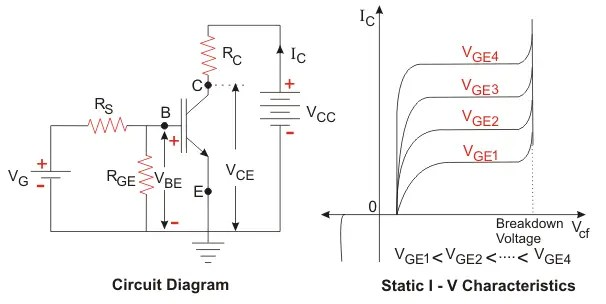# Why is Diode Non-Ohmic Semiconductor Device?

##### Difference Between Ohmic and non-Ohmic Conductors:Ohmic Conductors:
The conductors that obey the ohm’s law is called ohmic conductors. In an ohmic conductor, the current and the voltage has linear relationship. The current increase or decrease as the voltage is increased or decreased.The non-ohmic conductor has non-linear current-voltage relationship.

The resistance, inductance and the capacitor follows Ohm’s law.The current increase with an increase in the voltage.

Ohm’s Law:

At constant temperature, the current in a conductor is proportional to the voltage applied across the conductor.R= V/I
I=V/R

Where,
V= Applied Voltage
I  = Current in the circuit
R = Circuit Resistance

If the slope of the V-I graph is constant, the current and voltage has the linear relationship. The slope of the V-I graph can be constant only if the resistance of the circuit remain unchanged.

##### Non-Ohmic Conductors:

The non ohmic conductor does not follow the Ohm’s Law,  and the current drawn by the non ohmic conductor does not proportionately vary with the varying applied voltage.The resistance of non ohmic conductor does not remain constant when the voltage is applied across it. The changing resistance results in change in the current for a particular voltage and the V-I graph is no more linear.If we take the slope of  V-I curve of the  above graphs the resistor has the constant slope because of constant slope. The filament has variable resistance because the slope of the V-I graph is not constant.

All the semiconductor devices are non-linear devices because the current drawn by the devices is not proportional to the applied voltage. The semiconductor devices have a barrier potential and when the applied voltage is increased above the barrier potential the device starts conducting. If the voltage is increased above the barrier potential the voltage across the device remains constant and the current starts increasing. This happens because the concentration of the holes and electrons increases as the current through the device is increased. The resistance of the PN junction is not constant and does vary with the flow of electric current through the junction. The voltage and current has non linearity in the semiconductor junction diode.

Almost all the semiconductor devices which have nonlinear V-I relationship are as depicted below.

Where,
Io= Saturation Current

q= Charge of an electron- 1.6 *1019  Coulomb
K=Boltzmann Constant- 1.38 * 10– 23  JK1
T= Temperature(K)

The diode current increases exponentially when the voltage is increased above a particular value.
Similarly, the V-I characteristics of  silicon control rectifier(SCR) is also non-linear.The non-linear characteristics of the devices produce harmonics in the system. The non-linearity in the current with applied voltage cause harmonic distortion and other problems in the electrical network. The Insulated Gate Bipolar Transistors (IGBTs) are widely used in variable frequency drives,uninterrupted power supply(UPS). The IGBT has non-linear V-I characteristics.The collector current does not vary proportionately with an increase in the  collector to emitter voltage.